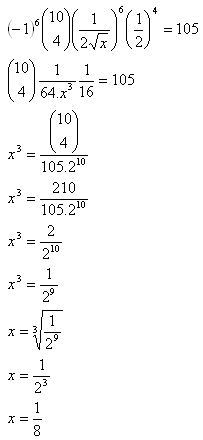sk|cz|

# Binomial Theorem

1.Express the Binomial Theorem and describe it‘s properties:

Binomial Theorem and the Pascal‘s Triangle.
Binomial Theorem
For each a, b є R, n є N stands: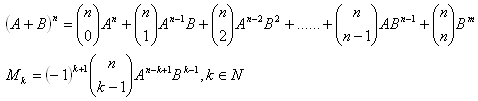Pascal‘s Triangle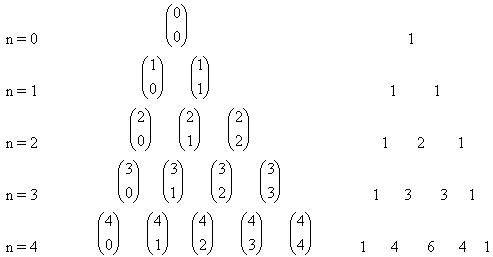Stands: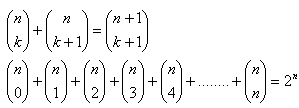2. Simplify: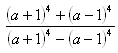Solution: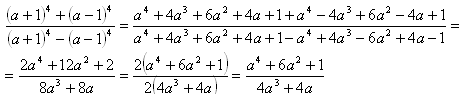3. Simplify: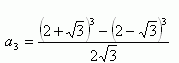Solution: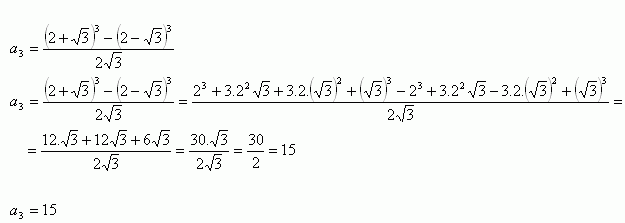4.Find out the fourth member of following formula after expansion: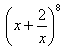Solution: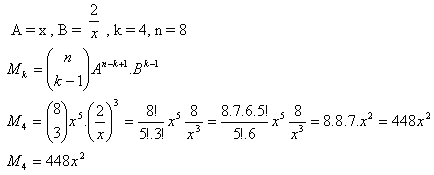5. Find out the member of the binomial expansion of ( x + x-1)8 not containing x.

Solution: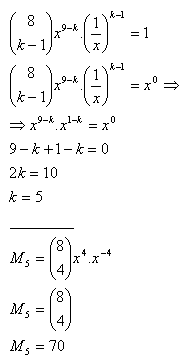The result is the number M5 = 70.

6. Which member of the binomial expansion of (2x3 + x–1)10 constains x6?

Solution: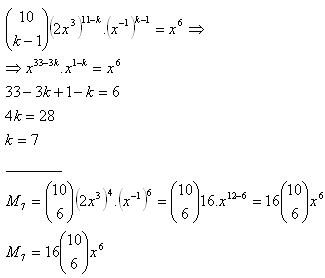7. In the expansion of (a + 2a3)n is the coefficient of the 3. expansion member greater by 44 than the 2. member‘s coefficient.
Find out a positive integer meeting these conditions.

Solution: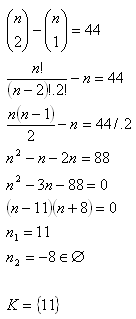8. For which x the 5th expansion member equals M5 = 105?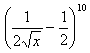Solution: